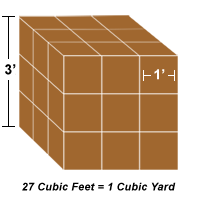### How it Works

### Stone and Mulch Calculators

As an example, let's use 500 square feet to be mulched at a
3" depth.

1. First, the depth must be converted to feet. To convert inches to feet, simply divide inches by 12, the number of inches in a foot.

So for our example: 3 inches divided by 12 equals a 0.25 foot depth.

2. Now that we have the depth in feet, we can multiply the depth by the area.

So for our example: 0.25 foot depth times 500 square feet equals 125 cubic feet.

3. If you are calculating 0.5, 2, or 3, cubic foot bags, simply divide 125 cubic feet by the size of the bag.

So for our example: if we are using 2 cubic foot bags, we can divide 125 cubic feet by 2 which equals 62.5 bags.

4. If you are calculating cubic yards, we need to convert 125 cubic feet to cubic yards by dividing by 27. One cubic yard is equal to 27 cubic feet, see the diagram below.So for our example: 125 cubic feet divided by 27 equals 4.63 cubic yards.